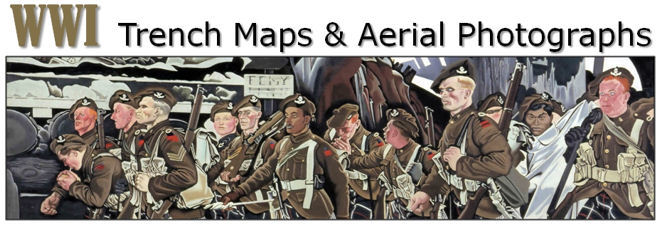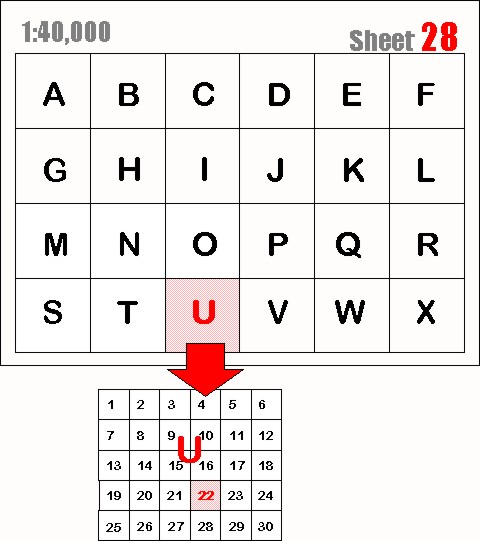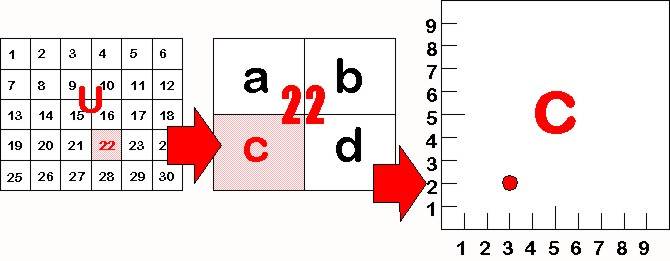How to Read Map Reference Numbers: The diagram below represents the grid on a typical World War I map at a scale of 1:40,000. It is divided into 24 lettered squares labelled A to X. We will use the following map reference number as an example: 28.U.22.C.3.2. The first number in the reference pertains to the map sheet number "28". Note: sheet numbers can also contain letters, as in sheet number "36cNW1" at 1:10,000 scale. see: scale. Next, follows the first letter which refers to the grid square "U" on that sheet. Each lettered grid square is then divided into smaller squares numbered from 1 to 30 (in some cases 1 to 36). In this example, the square number "22" is indicated in the map reference.This square 22 is then further divided into 4 squares labelled "a", "b", "c" & "d". In this example we have used the letter "C". Finally, square U.22.C. can be even further divided into tenths. On a 1:10,000 scale map you will actually see small division lines like those in the diagram below. The first number is read from left to right. In this example the number is "3" so, our reference point is 3 tenths from the left. Our second number is read from the bottom of the square up. In this example it is a "2" so, our point is also 2 tenths up from the bottom.Contact: mapref@mcmaster.ca